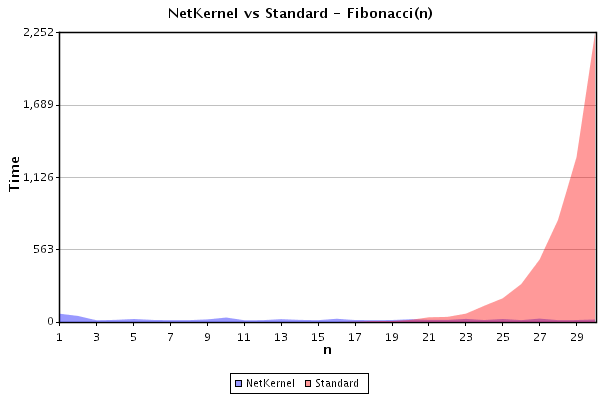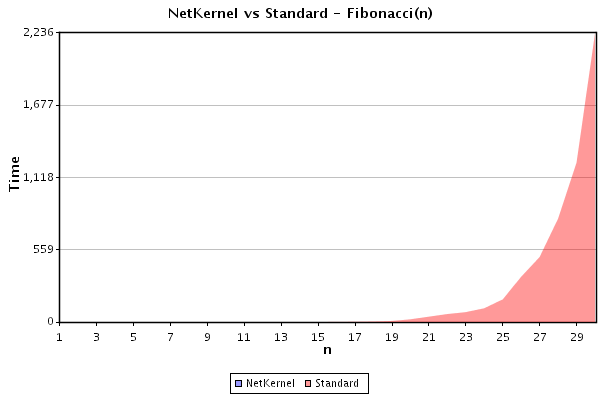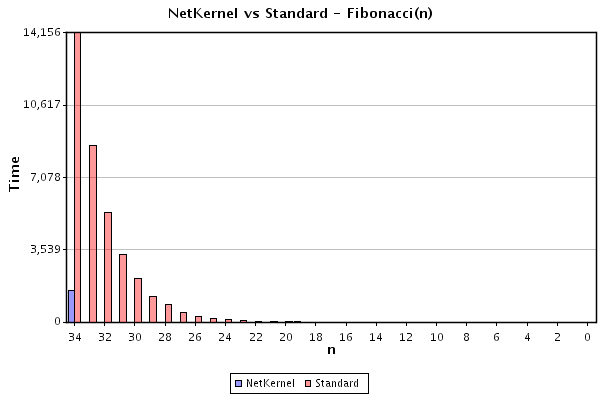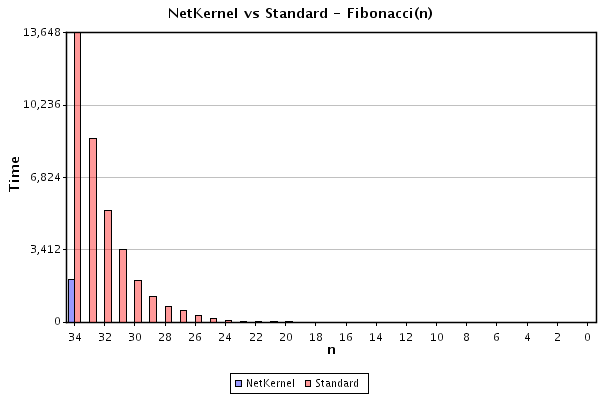# DRAFT Fibonacci Double Recursion Algorithm : A relative comparison of Procedural vs Resource Oriented Computation

## Peter Rodgers, Tony Butterfield.

### Introduction

This working draft presents a set of experimental results comparing the performance of the Fibonacci double recursion algorithm implemented as a 'standard' procedural function and as a NetKernel service located in a computational address space. This paper is a working draft report.

The aim of the experiment was to use a tightly constrained problem to demonstrate operating properties of the NetKernel system. It is not the intention of the authors to suggest that low-level algorithms yield higher performance in the NetKernel abstraction. Rather, we wished to show how the the empirical performance evidence we see in complex dynamically linked NetKernel business systems can be identified and be measured by using a constrained problem domain.

The experiment was performed on a NetKernel Standard Edition v3.1 system running on Java 1.4.2 with 64MB of heap.

## Experiment

The fibonacci double recursion algorithm is well known. Implemented in JavaScript it is:

```function fib(n)
{    if(n<2)    return n;
else
{    return fib(n-1)+fib(n-2);
}
}
```

For any value of n it computes the corresponding number in the Fibonacci sequence:

```n=0, 0
n=1, 1
n=2, 1
n=3, 2
n=4, 3
n=5, 5
n=6, 8
```

### Method

The fib(n) algorithm was implemented in JavaScript both as a standard native recursive JavaScript function (shown above) and as a recursive NetKernel service. The NetKernel service was located in a URI address space with logical address:

```active:javascript+operator@ffcpl:/fibonacci/fib-netkernel.js
```

The service takes one additional active URI argument 'n'. Where n is the recursion index. The NetKernel implementation, written in JavaScript, of the fib(n) algorithm is as follows:

```function fib(n)
{    if(n<2)    return n;
else
{   n1=context.sourceAspect("active:javascript+operator@ffcpl:/fibonacci/fib-netkernel.js+n@"+(n-1),IntegerAspect).getInt();
n2=context.sourceAspect("active:javascript+operator@ffcpl:/fibonacci/fib-netkernel.js+n@"+(n-2),IntegerAspect).getInt();
return n1+n2;
}
}
```

Here the NetKernel Foundation API method sourceAspect() is a recursive call within the NetKernel address space.

The NetKernel implementation uses an IntegerAspect (a simple value object to hold an int) as its resource model which is constructed and returned as a response for each recursion. For each invocation it must parse the requesting URI in order to obtain the 'n' index value - this step is not shown. In addition the parsed n value is converted to and from string/integer within the algorithm incurring an additional overhead compared to the standard implementation.

Both the NetKernel and the standard implementations were executed on the JavaScript engine inside NetKernel. The experiment aims to provide a relative performance comparison, therefore by implementing both approaches with the same language the performance characteristics of the language are removed as a factor. Furthermore, on NetKernel, JavaScript is dynamically compiled to Java bytecode for execution, therefore the local fib(n) implementation is a very tight recursive function when expressed as Java bytecode.

The two implementations were run back-to-back in a controlling benchmark script which timed the total execution time of each implementation. The inner timing loop is as follows:

```var n=0;
while(n<30)
{    n=n+1;

//NETKERNEL
t1=System.currentTimeMillis();
f1=context.sourceAspect("active:javascript+operator@ffcpl:/fibonacci/fib-netkernel.js+n@"+n, IntegerAspect).getInt();
t2=System.currentTimeMillis();
times.* += <test><method>nk</method><time>{t2-t1}</time><n>{n}</n><fib>{f1}</fib></test>;

//STANDARD
t1=System.currentTimeMillis();
f2=fib(n);
t2=System.currentTimeMillis();
times.* += <test><method>standard</method><time>{t2-t1}</time><n>{n}</n><fib>{f2}</fib></test>;
if(f1!=f2)
{ 	System.out.println("INVALID RESULT AT STEP"+n);
break;
}
}
```

The standard fib(n) function (denoted as 'Standard' in the results) is implemented in the local benchmark script and therefore has the advantage of very tight local execution on a single thread with no kernel scheduler overhead and has no type conversion overhead.

Results and computation times are accumulated in an E4X XML variable.

## Results

### Result 1: Forward computation of the sequence n=1 to n=30

The benchmark script was run 'forward' computing fib(n) for both implementations with n progressively incremented from 1 to 30. In a clean-system with an empty cache, for each value of n, fib(n) was computed; first, by requesting the NetKernel service, and second, by locally invoking the standard procedural function. The time in milliseconds taken to compute fib(n) for each implementation is presented in figure 1:As is well known, the standard algorithm computation time grows exponentially rapidly. By contrast the NetKernel implementation executes in constant time. NetKernel becomes faster than the standard algorithm at approx n=18.

The NetKernel implementation displays a small amount of scheduler 'noise'. The requests for recursive execution are made asynchronously and are placed in the NetKernel Scheduler to await scheduling. The NetKernel system was not tuned in any way and was a regular production Standard Edition v3.1 system. Therefore normal system processes (eg cron) are competing with the recursion experiment for Scheduler time.

It is interesting to note that this result hints at the intrinsic system tuning of NetKernel. It is seen that the first execution n=1 time for the NetKernel result is slightly longer than the others. This is explained since the first call to the JavaScript service must incur the cost of the initial loading time of the JavaScript code and its transreption (compilation) to bytecode. In the standard fib(n) call, the JavaScript load and compile has already occured before starting the timing loop and any internal fib(n) calls are made.

### Result 2: Repetition of the forward computation

The benchmark script was immediately re-run performing a repeat of the 'forward' computation. Results for n progressively incremented from 1 to 30 are shown in figure 2:It can be seen that the results for the standard algorithm are the same. By contrast the NetKernel implementation computes the values instantly (below the 1ms resolution of the Java system timer).

This result demonstrates that the results of the forward computation shown in Result 1 remain cached in the NetKernel system cache. A subsequent execution of the benchmark test finds and retrieves each NetKernel service fib(n) result from the cache. The result demonstrates that resolving a computation value in the space of existing computed values is very cheap and that if a result exists the algorithm computation cost is effectively zero.

### Result 3: Backward computation n=34 to n=0

The benchmark script was run in reverse from n=34 to n=0. The system was clean with an empty cache.

The results are shown in figure 3:It can be seen that the standard algorithm performs as before. By contrast NetKernel is substantially faster at computing fib(34) and each subsequent computation of fib(n) for n<34 is instantaneous (below the resolution of the timer).

This result is initially quite suprising. In the previous results (1,2) one can intuitively see that previously computed fib(n) resources are accumulated in the cache and so each step of n can take advantage of the previous steps computational trail. When the computation is performed in reverse, no previous values of fib(n) are available in the computation address space therefore the NetKernel implementation must recursively generate each fib(n).

We must mentally unravel the recursion in order to understand the result. The double recursion in the algorithm effectively has two sides: first the fib(n-1) branch of the recursion tree is walked down to n=0, this side then unwinds returning n-1, but at each return of fib(n-1), the second side of the algorithm must request fib(n-2), however each time this value is requested it is discovered that the first pass has already populated the space with the result. Hence most of the branches of the recursion tree do not require evaluation. We show a detailed analysis of this result below.

## Energy Analysis

We shall now consider the energy costs of the computations performed in the experiment. We shall consider relative energy explicitly since by doing so we are able to make a direct comparison between the standard computation and the results obtained by accessing the resource oriented computation environment. Traditional O notation algorithm analysis is confined to the intrinsic world of the algorithm and therefore cannot know the extrinsic properties provided by the NetKernel computational address space.

First we shall consider the standard local approach and then compare this with the NetKernel based extrinsic approach.

### Standard

The standard local double recursion function is:

```         {     n                         (n < 2)
fib(n) = {
{     fib(n-1) + fib(n-2)       (n >= 2)
```

α, the computation energy cost of each call to the fib(n) procedure is constant. Therefore we can express the energy cost of fib(n) as:

e0 = α

e1 = α

e2 = α + e1 + e0 = 3 α

en = α + en-1 + en-2

It follows that the energy cost to evaluate fib(n) using the local double recursion algorithm is:

En = (2 fib(n + 1) - 1) α             

The energy expenditure is proportional to the fibonacci number and is exponential in n.

### NetKernel (Forward)

Let's now analyze the NetKernel implementation of the forward computation of n=0..30 shown in result 1. As in the local implementation, the NetKernel fibonacci algorithm is:

```         {     n                         (n < 2)
fib(n) = {
{     fib(n-1) + fib(n-2)       (n >= 2)
```

However in the NetKernel implementation fib(n) is located in a computational space and each call to fib(n) is made through the space. Therefore we must consider two energies: α', the energy cost of a kernel-mediated call through the address space to invoke the JavaScript code (it is clear that, α' > α ). In addition we must consider: β, the energy cost of resolving a predetermined resource value in the space (a cached computational resource).

We can express the energy cost of fib(n) for n monotonically increasing as:

e0 = α'

e1 = α'

e2 = α' + 2 β

en = α' + 2 β

Therefore the total energy cost of each step is constant. In order to compare with the total local energy E (shown in ), we can calculate the cumulative energy cost E' as follows:

E'n = Σi=0n ei

Which it can be shown gives:

E'n = (n + 1) α' + 2 (n - 2) β

If (as is the observed case in the rerun results 2), β << α' then we have:

E'n = (n + 1) α'             

Therefore NetKernel linearizes the double recursion algorithm.

### Breakeven Point

It is interesting to consider the energy breakeven point observed in result 1. NetKernel becomes faster than the local method at about 18 < n < 19. From  and  we can equate the energies and obtain the ratio of the α energies as:

α'        2 fib(n + 1) - 1
__ = _____________             
α            n + 1

From which we find that α'/α ~ 400 for our observed breakeven between n=18 and n=19. That is, the relative energy costs of a local vs mediated NetKernel execution is approximately 400 or, put another way, the entire NetKernel address space abstraction costs only 400 times that of a single Java bytecode function call and is constant irrespective of the local computation cost for a resource in the space. Recall that this is an unoptimized system and that α' includes the costs of computing and parsing the request URI value and of marshalling and unmarshalling and type conversion of the computed resources and so represents a worst case. Without these costs we estimate that the ratio would be of the order of 10s rather than 100s - that is, the NetKernel abstraction cost is roughly constant and actually very slight compared with an intrinsic 'traditional' solution. Future work with our latest Kernel implementation will yield a more fair comparison.

### NetKernel (Reverse)

To complete the analysis let's consider result 3. Running NetKernel in reverse from 34 to 0 with an initially empty cache. Here finding the energy cost is slightly more complicated since the algorithm must recurse since the address space (cache) is initially empty. Furthermore, as the algorithm progresses it starts to discover precomputed results in the adddress space. By expanding the recursion for some values of n, we see that:

E'4 = α' + [ α' + [α'] + [α'] + [α'] + β ] + β = 5 α' + 2 β

Equally

E'5 = α' + [α' + [ α' + [α'] + [α'] + [α'] + β ] + β] + β = 6 α' + 3 β

Therefore by induction we obtain:

E'n = (n + 1) α' + (n - 2) β      (n > 1)

And for β << α' :

E'n = (n + 1) α'      (n > 1)             

Again we observe that within NetKernel the double recursion fib(n) is linear in n. It follows that  represents an upper bound on the energy cost to compute fib(n) in the NetKernel address space and when we consider that the computation space may include existing accumulated computational history then we can assert that the total must always lie within the limits:

E'n <= (n + 1) α'                
E'n >= β                             

## Discussion

By locating a computation and the computed resource within a computational address space we are able to accumulate computational knowledge. It is our empirical observation that computational resources, especially those in business applications, have an enduring lifetime and hence acquire a reusability that is perhaps surprisingly valuable. In the fib(n) double recursion experiment we observe in a very tightly coded algorithm that the properties of a computational address space often act so as to minimize system computational energy costs.

In order to better understand what is happening in a computation in NetKernel it is helpful to think in terms of bounded infinite computation spaces. So, for example in this experiment, the base location of the fib(n) URI is:

```active:javascript+operator@ffcpl:/fibonacci/fib-netkernel.js
```

which is the bounded infinite set (space) of all possible fibonacci computations. When we request a value from the space by issuing a URI request such as:

```active:javascript+operator@ffcpl:/fibonacci/fib-netkernel.js+n@30
```

then NetKernel computes the value. However in NetKernel 'computing the value' might mean that that the point in the space is already known (it is cached under that URI) and so requires no (or very very little) computation energy expenditure. By storing computational state NetKernel is trading algorithm execution time with space.

### Memoization and the NetKernel Realization

Storing and reusing computed values of a function is not new, in the literature it is called Memoization, and is related to, but qualitatively different from, caching. Some languages such as Lisp, Haskell, Scheme and Lua support memoization as a feature. Memoization is usually implemented by making an explicit modification to a function - though some languages (Lua) permit memoization transparently. We have shown above, that NetKernel is a computing environment that provides automatic and transparent memoization.

Since NetKernel's computational address space resides outside any given language then NetKernel offers a generalization of memoization, lifting it out of the language domain and into the computational abstraction. We term NetKernel's generalization of memoization, Realization since the NetKernel computing abstraction regards computation as the reification (physical realization) of a representation of an abstract information resource - the set of physically realized information resources is then termed "The Realization".

To show that the NetKernel Realization resides outside of the language domain. We constructed a further experiment which splits the fib(n) function into two: a Beanshell (scripted Java) version for odd n and a JavaScript version for even n. In order to obtain a value of fib(n) we must therefore alternately call both odd and even implentations to obtain fib(n-1) and fib(n-2). Therefore, the fib(n-odd) resources are in one space and fib(n-even) are in another space. It is unsurprising that the results for computing fib(n) in this heterogeneous space are the same as before. Below is a repeat of result 3, computed half in beanshell and half in javascript:Traditionally, in order to replace a function call with a precomputed value the memoized function must be referentially transparent. That is, it must always return the same value for the same inputs - in other words, it must not use any external scope, such as global variables. In NetKernel, the address space exists independently and outside of any given language. Since NetKernel is mediating the use of all resources in the space, it is able to assert referential transparency independently for any function in the space. In future work we will discuss how NetKernel's address space abstraction is a generalization of variable scope within a language into a generalized extrinsic spacial computational context.

In a further refinement to memoization, NetKernel accumulates a computational cost (Thread CPU time /Energy) and dependency metadata for each stored computational resource. This information is readily used to obtain a figure of merit to determine the relative system-wide value of holding a resource in memory. Computational resources which are infrequently used or whose dependent members have expired are eventually purged from memory. Therefore NetKernel's extrinsic managed computational space allows a balanced energy/space trade-off to be made and permits the system-wide energy cost to reside in a dynamic local minima.

### Scale and complexity

The whole experiment demonstrates that NetKernel's abstraction permits the accumulation of long-lived computational resources. In ordinary computing systems this information is thrown away as a side-effect. As is shown in Result 3, computational information is often reusable and can dramatically impact subsequent overall system performance.

The experiment was a deliberately constrained problem - in many ways it benefits from the naivity of implementation of the double recursion algorithm. We would not expect an NP-complete problem to show such dramatic effects since, when an NP-complete problem is considered in the computational space abstraction, we understand that the boundary of unknown computational resources grows exponentially faster than the accumulation of knowledge of any sub-set of the space. However, what we are observing is nonetheless a dramatic effect, NetKernel is 'serendipitously' linearizing the exponential cost of the naive fib(n) algorithm.

As we stated in the introduction. It is not our thesis that NetKernel should be considered for replacing small locally coded algorithms - though it is interesting to speculate on which types of algorithm may benefit most from an extrinsic computational space. Rather, we performed this controlled analysis in order to offer a firm handle onto our daily observation of macroscopic energy minimization in large-scale complex applications.

As is the nature of business applications, there is almost never a single point at which one could systematically perform algorithmic analysis nor even practically seek to find global systemic optimization. However even given the practical economics of the real-world, our observation is that NetKernel continually and dynamically finds local energy minima in the computational space of such applications and exploits the innate and often serendipitous optimization inherent in resource oriented computation.

## References

 NetKernel Standard Edition v3.1 http://www.1060research.com Source code and installable Fibonacci Module http://resources.1060research.com/docs/fibonacci.jar Memoization http://en.wikipedia.org/wiki/Memoization